Home Practice
For learners and parents For teachers and schools
Textbooks
Full catalogue
Pricing SupportLog in

We think you are located in United States. Is this correct?

# End of chapter exercises

## 12.8 End of chapter exercises

Exercise 12.8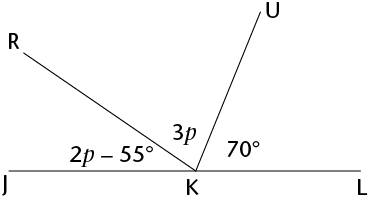Calculate the size of:

1. $$p$$
2. $$R\hat{K}U$$
1. \begin{align} 2p-55°+3p+70° &=180° &(\angle\text{s on a straight line}) \\ 5p+125°&=180° \\ 5p&=55° \\ p&=11° \end{align}
2. $R\hat{K}U = 3p =3(11^{\circ})=33^{\circ}$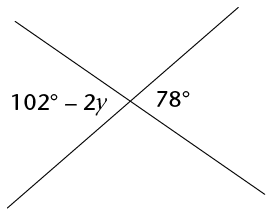Calculate the size of $$y$$

\begin{align} 102°-2y &=78° & (\text{vert opp }\angle\text{s}) \\ -2y &=78°-102° \\ -2y &=-24° \\ y &= 12° \end{align}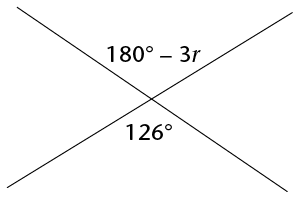Calculate the size of $$r$$

\begin{align} 180°-3r &=126° & (\text{vert opp }\angle\text{s}) \\ -3r &=126° - 180° \\ -3r &=-54° \\ r &=18° \end{align}

Find the sizes of all the angles $$\hat{1}$$ to $$\hat{7}$$.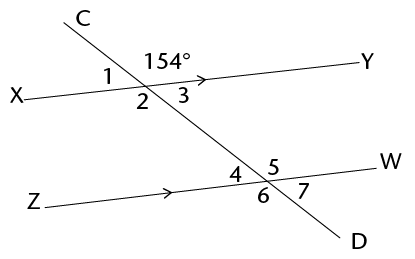\begin{align} \hat{1} &= 180°-154°&&(\angle\text{s on a straight line}) \\ \hat{1} &= 26° \\ \hat{2}&=154°&& (\text{vert opp }\angle\text{s}) \\ \hat{3}&=26°&& (\text{vert opp }\angle\text{s}) \\ \hat{4} &=\hat{1} &&(\text{corresp }\angle\text{s}; XY \parallel ZW) \\ \hat{4} &= 26° \\ \hat{5} &=154 °&&(\text{corresp }\angle\text{s}; XY \parallel ZW) \\ \hat{6}&=154°&& (\text{vert opp }\angle\text{s}) \\ \hat{7}&=26°&& (\text{vert opp }\angle\text{s}) \\ \end{align}

In the diagram, $$OK = ON$$, $$KN \parallel LM$$, $$KL \parallel MN$$ and $$\hat{LKO}=160^{\circ}$$. Calculate the value of $$x$$. Give reasons for your answers.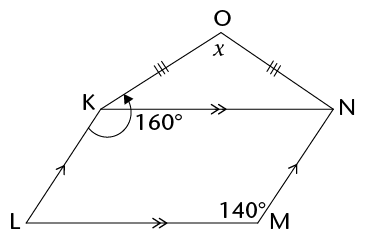\begin{align} \hat{LKN} &=140° &&(\text{opp }\angle\text{s} \text{ of parallelogram = )} \\ \hat{OKN} &=160°-140° \\ \hat{OKN} &=20° \\ \hat{ONK} &=20° &&(\angle\text{s opp = sides}) \\ \hat{ONK}+ \hat{OKN}+ x &=180°&&(\text{sum of }\angle\text{s in } \triangle ) \\ 20°+ 20° +x &=180° \\ x&=180° -20°-20° \\ x&=140° \\ \end{align}